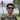## Akhila Ariyachandra# Data Structures: Stack

April 22nd, 2020

Stack is a linear data structure where the elements follow a First In Last Out (FILO) order. It can also be thought as Last In First Out.

Stacks can be used for things like tracking which functions are currently running and the navigation state in a web app.

## Implementing a Stack data structure

Even though we will be implementing the Stack data structure in TypeScript in this example, the basic concept can be implemented in any language.

First declare a class.

``class Stack<T> {}``

Then declare a private member variable array to store the data of the stack. Making it private will stop it from being directly accessed from outside the class.

``````class Stack<T> {
private _data: T[] = [];}``````

Next, we need a way to check the size of the stack. We can use a getter to access the size of the `_data` array.

``````class Stack<T> {
// ...

// Check the size of the stack  get size(): number {    return this._data.length;  }}``````

After that we’ll add a method to check whether the stack is empty or not.

``````class Stack<T> {
// ...

// Returns whether the stack is empty or not  public isEmpty(): boolean {    return this._data.length === 0;  }}``````

Now we need a method to add new elements to the stack. We can use the `push()` method in arrays to add an element to the end of the `_data` array.

``````class Stack<T> {
// ...

// Adds an element to the top of the stack  public push(element: T): T {    this._data.push(element);    return element;  }}``````

Finally we will implement two methods to check the element on top of the stack. One will just check the value, `peek()`, and the other will remove the element from the stack and return it, `pop()`. Both will throw an error if they are called when the stack is empty, i.e. the length of the `_data` array is zero.

``````class Stack<T> {
// ...

// Returns the value of the element on top of the stack without removing it  public peek(): T {    if (this.isEmpty()) {      throw new Error("Stack is empty");    }    return this._data[this._data.length - 1];  }  // Removes the topmost element of the stack and returns it  public pop(): T {    if (this.isEmpty()) {      throw new Error("Stack is empty");    }    return this._data.pop() as T;  }}``````

Finally your stack class should look like this.

``````class Stack<T> {
private _data: T[] = [];

// Check the size of the stack
get size(): number {
return this._data.length;
}

// Returns whether the stack is empty or not
public isEmpty(): boolean {
return this._data.length === 0;
}

// Adds an element to the top of the stack
public push(element: T): T {
this._data.push(element);

return element;
}

// Returns the value of the element on top of the stack without removing it
public peek(): T {
if (this.isEmpty()) {
throw new Error("Stack is empty");
}

return this._data[this._data.length - 1];
}

// Removes the topmost element of the stack and returns it
public pop(): T {
if (this.isEmpty()) {
throw new Error("Stack is empty");
}

return this._data.pop() as T;
}
}``````

Below is an example on how to use the Stack class we just created. The stack can be created to hold any type of data.

``````const numberStack: Stack<number> = new Stack<number>();

console.log(numberStack.isEmpty()); // true
console.log(numberStack.size); // 0

numberStack.push(1);
// 1
numberStack.push(2);
// 1, 2
numberStack.push(3);
// 1, 2, 3

console.log(numberStack.isEmpty()); // false
console.log(numberStack.size); // 3

console.log(numberStack.peek()); // 3
console.log(numberStack.pop()); // 3
console.log(numberStack.pop()); // 2
console.log(numberStack.pop()); // 1

console.log(numberStack.isEmpty()); // true
console.log(numberStack.size); // 0``````